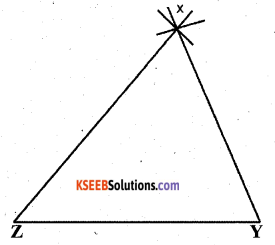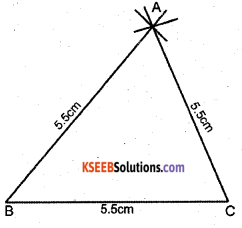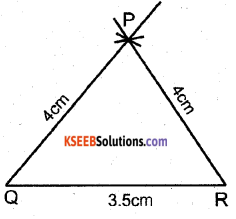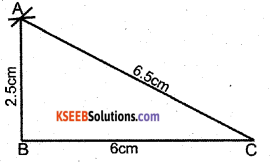# KSEEB Solutions for Class 7 Maths Chapter 10 Practical Geometry Ex 10.2

Students can Download Chapter 10 Practical Geometry Ex 10.2, Question and Answers, Notes Pdf, KSEEB Solutions for Class 7 Maths, Karnataka State Board Solutions help you to revise complete Syllabus and score more marks in your examinations.

## Karnataka State Syllabus Class 7 Maths Chapter 10 Practical Geometry Ex 10.2

Question 1.
Construct ∆ XYZ in which XY = 4.5 cm, YZ = 5 cm and ZX = 6 cm.
Solution:Steps of Construction

1. Draw a line segment YZ of length 5 cm with Y as centre, draw an arc of radius 4.5 cm.
2. With Z as centre, draw an arc of radius 6cms which cuts the first arc. Mark the point of intersection of arcs as X.
3. Join XY and XZ. ∆ XYZ is the required triangle.Question 2.
Construct an equilateral triangle of side 5.5 cm.
Solution:
Steps of Constructions1. Draw a line segment BC of length 5.5 cms. with ‘B’ as centre draw an arc of radius 5.5 cm.
2. With ‘C’ as centre, Cut the first arc with radius 5.5 cms. Mark the point of intersection of arcs as ‘A’
3. Join AB and AC. ∆ ABC is the required equilateral triangle.

Question 3.
Draw ∆ PQR with PQ = 4 cm, QR = 3.5 cm and PR = 4 cm. What type of triangle is this ?
Solution:Steps of Construction

1. Draw a line segment QR of length 3.5 cm with Q as centre, draw an arc of radius 4 cm.
2. With R as centre, cut the first arc with a radius 4 cm. Mark the point of intersection of arcs as P.
3. Join PQ & PR A PQR is an isosceles triangle PQ = PR.Question 4.
Construct ∆ ABC such that AB = 2.5 cm, BC = 6 cm and AC = 6.5 cm. Measure <B.
Solution:
Steps of Construction

1. Draw a line segment BC of length 6 cm with ‘B’ as centre, draw an arc of radius 2.5 cm.
2. With ‘C’ as centre cut the first arc with a radius 6.5 cm.
3. Mark the point of intersection of arcs as A. Join AB & AC. ∆ ABC is the required triangle.